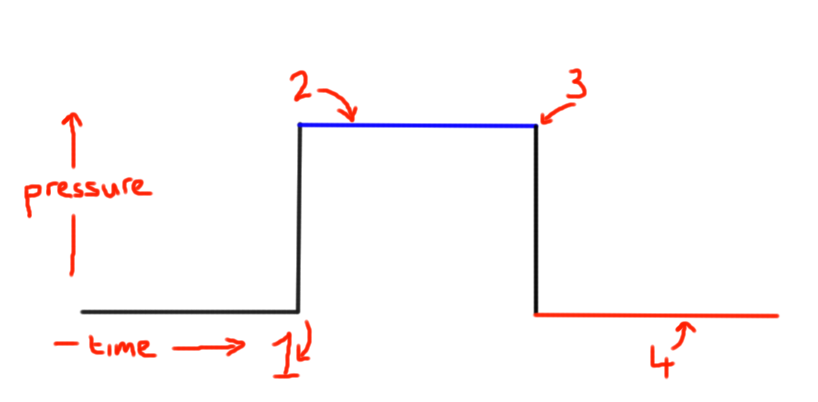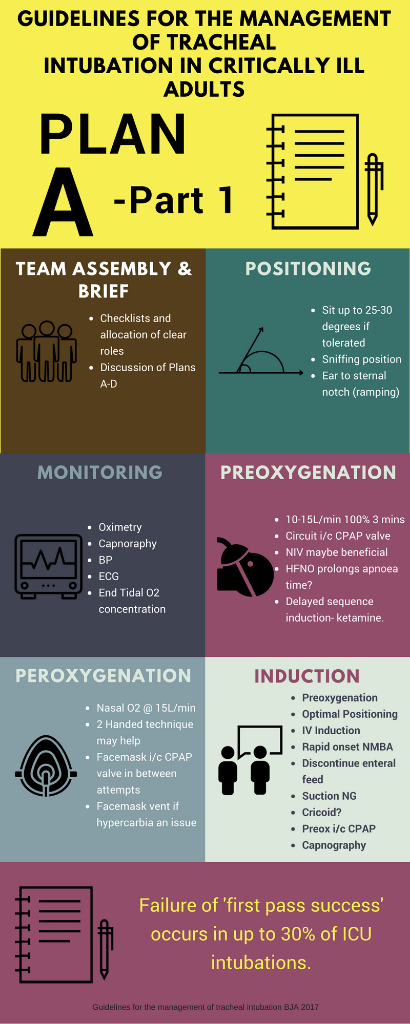Mechanical Ventilation- Phases of a breath- I:E ratio and total cycle time. - Critical Care Practitioner

CriticalCarePractitioner

## Mechanical Ventilation- Phases of a breath- I:E ratio and total cycle time.On the simple ventilator pressure waveform we have time on the x axis and pressure on the y axis. This is a square shaped waveform.

So on the first part of the waveform the pressure is at the baseline and nothing is happening, but then at (1) the pressure rises in a vertical fashion as air is entrained into the lung.

But what has happened to cause this to occur?

What has triggered the start of inspiration at (1)?

The inspiration phase is then at (2).

The breath then ends (3) at end inspiration.

Then we reach baseline or expiratory phase again (4).

This whole process takes place over a total cycle time, in other words how long does this whole breath take. The whole breath includes both the inspiration time, the expiration time and any pauses in between. In the diagram above the cycle starts at (1) and finishes at the end of (4).

So let us assume that the ventilator is giving 12 breaths every minute, a rate we can set. We need then to divide 60 seconds by 12 which will give us a total cycle time of 5 seconds.

From this we can work out how much of that total cycle time is inspiration and how much is expiration- this is the inspiration:expiration ratio or I:E ratio.

We can set our inspiratory time via the ventilator in many modes of ventilation. So let us assume that we set our inspiratory time or Ti to 1 second. So as our total cycle time was 5 seconds, we must have an expiratory time of 4 seconds. This will then give us an I:E ratio of 1:4.

So, in this example, we have four times as long in expiration as we do in inspiration.

So what will happen if we decrease the respiratory rate to 10 breaths per minute for example? This will mean that we now have 6 seconds per breath. If we don’t change the Ti, so that inspiration still takes 1 second then expiration will now take 5 seconds.

The I:E ratio has changed to 1:5. Expiration now takes five times longer than inspiration.